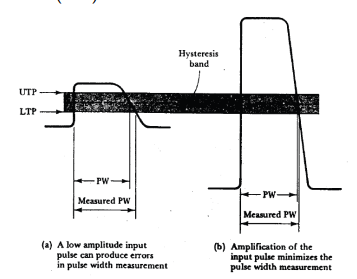### Basic Digital Frequency Meters (DFM)

The operation of basic DFM:

1. The input signal is amplified or attenuated as necessary.

2. The input signal is converted to a square wave and is fed to one terminal of the AND gate.

3. The time base signal with 1 Hz. freq. is fed to a flip-flop.

4. The flip-flop changes its state at each falling-edge of the time base. It divides the frequency by 2 giving a high on the Q terminal for 1 s and a low for another 1 s. The terminal Q¯ is an inverted version of Q.

5. One terminal of the AND gate is fed from the flip-flop Q output and the other terminal is fed from the shaped input signal. So, the counter circuit will count the input pulses for the duration of 1 s. (Frequency).

6. The counter will reset to zero at each negative (falling) edge of the Q .

7. The latch will isolate the counting from the display during the first 1 s and will update the display on the rising edge of Q .

Frequency Range Changing:

• Different time-base frequencies could be used to give several range of frequency measurements.

• The different time base could be achieved by connecting a series decade counter. Each decade counter divides the frequency by 10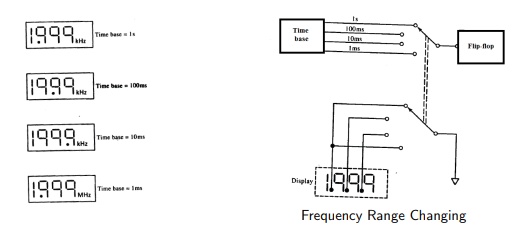Range Selection Error:

• The lowest possible frequency range should be used for the greatest measurement accuracy.

Frequency Meter Accuracy:

• Accuracy Specification There is always a possible error of ±1 cycle in the measured frequency due to the partial input pulse that may or may not succeed in triggering the counting circuit. This one cycle is defined as least significant digit (LSD).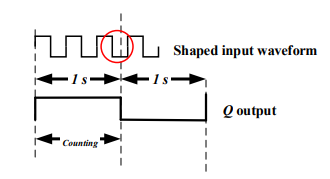• Accuracy Specification Also, the time base error Etb due to freq. variation will give a reading error in the measured frequency fi as:

time base error = fi x Etb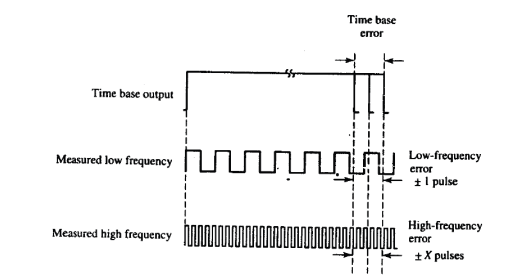So, the total accuracy of digital frequency meter is specified as:

±1 LSD ± time base error

## Reciprocal Digital Frequency Meters (DFM)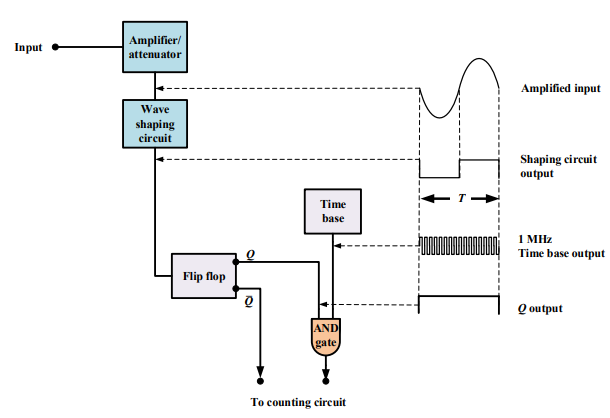• The time base signal with 1 MHz. is applied directly to the AND gate.

• The reshaped input signal is employed to toggle the flip-flop circuit.

• It is better for measuring low frequencies than the direct frequency meters. The measure frequency fin is:

• fin = ftime base/n

Time and Ratio Measurements:

• Frequency Ratio Measurement If the flip-flop in Reciprocal FM is made to toggle on +ve and -ve edges, we can measure the input pulse width.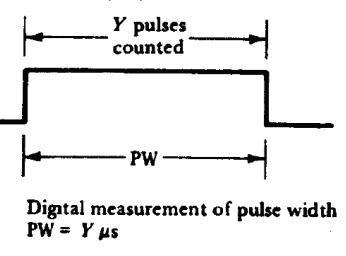Frequency Ratio Measurement:

• To find the ratio between two frequencies f1 and f2, the higher frequency signal is fed to the AND while the lower frequency is applies to the flip-flop.

Digital Frequency Input Stage:

Why we use the attenuator/amplifier in input ?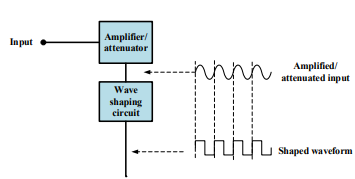In the case of noisy input signal, an error is exist due to the amplified signal and the amplified noise.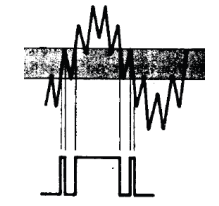To reduce the effect of noise, the input signal should be attenuated to attenuate the input noise.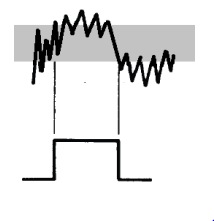UTP: Upper Triggering Point.

LTP: Lower Triggering Point.

The small input signal could be amplified to make it suitable to be triggered by UTP and LTP.

Also, amplification could help in reducing the measurement error due to hysteresis when measuring the Pulse Width (PW)